Home > AC > Chapter 11 > Lesson 11.1.6 > Problem11-73

11-73.
1. Solve for x in each equation below. Homework Help ✎

1. 2x = 8

2. 2x + 2 = 10

3. 6x + 2 − 4x = 10

4. 2(3x + 1) − 4x = 10

5. Check your solutions for the equations above. What do you notice?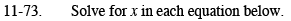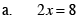Divide both sides by 2.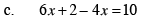Combine like terms on the left side of the equation. Then see part (b).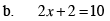Subtract 2 from both sides.Then see part (a).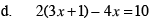Distribute the 2. Then see part (c).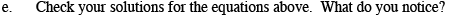All of your answers should be the same.